Welcome To Basics In Maths

# ICSE IX Class Maths Concept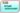# Math problems asked in the CBSE board and entrance examinations.

## 1. RATIONAL AND IRRATIONAL NUMBERS

Natural numbers: counting numbers 1, 2, 3… called Natural numbers. The set of natural numbers is denoted by N.

N = {1, 2, 3…}

Whole numbers: Natural numbers including 0 are called whole numbers. The set of whole numbers denoted by W.

W = {0, 1, 2, 3…}

Integers: All positive numbers and negative numbers including 0 are called integers. The set of integers is denoted by I or Z.

Z = {…-3, -2, -1, 0, 1, 2, 3…}

Rational number: The number, which is written in the form of, where p, q are integers and q ≠ o is called a rational number. It is denoted by Q.

∗ In a rational number, the numerator and the denominator both can be positive or negative, but our convenience can take a positive denominator.

Ex: –can be written asbut our convenience we can takeEqual rational numbers:

For any 4 integers a, b, c, and d (b, d ≠ 0), we have⇒ ad = bc

The order of Rational numbers:

If  are two rational numbers such that b> 0 and d > 0 then⇒ ad > bc

The absolute value of rational numbers:

The absolute value of a rational number is always positive. The absolute value ofis denoted by.

Ex: – absolute value ofTo find rational number between given numbers:

• Mean method: – A rational number between two numbers a and b isEx: – insert two rational number between 1 and 2

1 << 2   ⟹     1 << 2

1 << 2   ⟹   1 <2

To rational numbers in a single step: –

Ex:- insert two rational number between 1 and 2

To find two rational numbers, we 1 and 2 as rational numbers with same denominator 3

(∵ 1 + 2 = 3)

1 =and 2 =Note: – there are infinitely many rational numbers between two numbers.

The decimal form of rational numbers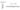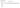∗ Every rational number can be expressed as a terminating decimal or non-terminating repeating decimal.

Converting decimal form intoform:

1.Terminating decimals: –

1.2 =1.35 =2.Non-Terminating repeating decimals: –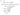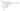Irrational numbers:

• The numbers which are not written in the form of, where p, q are integers, and q ≠ 0 are called rational numbers. Rational numbers are denoted by QI or S.
• Every irrational number can be expressed as a non-terminating decimal or non-repeating decimal.

Ex:-and so on.

• Calculation of square roots:
• There is a reference of irrationals in the calculation of square roots in Sulba Sutra.
• Procedure to findingvalue: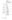#### Visit My Youtube Channel:  Click  on below  logo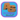error: Content is protected !!